# 多智能体强化学习算法

## From Few to More：Large-scale Dynamic Multiagent Curriculum Learning

Posted by MY on August 14, 2020

## 一、问题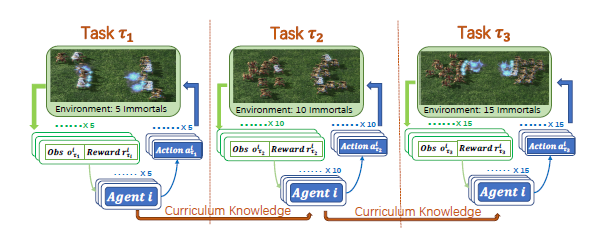## 二、解法

### 2.1 Partially Observable Stochastic Games

Partially Observable Stochastic Games（POSG）是MDP在多智能体设置下的自然扩展，定义为$\left\langle{\mathcal{N}}, \mathcal{S}, \mathcal{A}^{1}, \cdots, \mathcal{A}^{n}, T, \mathcal{R}^{1}, \cdots, \mathcal{R}^{n}, \mathcal{O}^{1}, \cdots, \mathcal{O}^{n}\right\rangle$：

• $\mathcal{N}$：n个智能体
• $\mathcal{S}$：状态集合
• $\mathcal{A}^{i}$：智能体$i$的动作集合，$\mathcal{A}=\mathcal{A}^{1} \times \mathcal{A}^{2} \times \cdots \times \mathcal{A}^{n}$是联合动作空间。
• $T$：转移函数
• $\mathcal{R}^{i}$：智能体$i$的奖励
• $\mathcal{O}^{1}$：智能体$i$的观察，$\{o_{t}^{i, e n v}, m_{t}^{i}, o_{t}^{i, 1}, \cdots, o_{t}^{i, i-1}, o_{t}^{i, i+1}, \cdots, o_{t}^{i, n}\}$是智能体$i$在时间$t$的观察，其中$o_{t}^{i, e n v}$是对周围环境的描述；$m_{t}^{i}$是智能体的私有性质，如位置、健康状态等；$o_{t}^{i, i-1}$是智能体$i$对智能体$i-1$的观察，如两个智能体之间的相对位置等。

### 2.2 大规模多智能体系统的性质

• 部分可观察性：在MAS中，智能体根据他们自己的观察来做出决策，从而可以将大规模问题减少为相对独立但又相关的子问题。
• 稀疏交互：从全局角度来看，在同一时刻，每个智能体仅与MAS中的某些智能体交互，并且这种交互并非始终发生。
• 状态语义：每个状态都包含语义信息，可用于衡量状态之间的相似性。例如，在《星际争霸2》中，随着游戏的进行，如果士兵的任何一方在战斗中死亡，智能体的数量将会减少，这种情况下的学习与小规模战斗中的学习类似。

### 2.3 知识迁移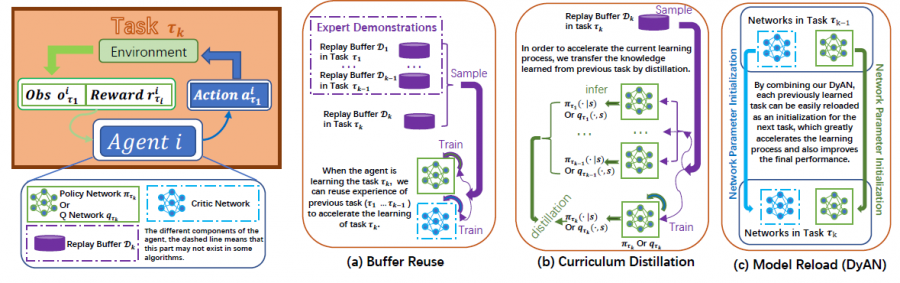• Buffer Reuse
• Curriculum Distillation

#### 2.3.1 Buffer Reuse

$\text { Loss }=\sum_{i=1}^{k} \sum_{j=1}^{b}\left[\left(r_{i}^{j}+\gamma \max _{a_{i}^{\prime} j} q_{\tau_{i}}\left(s_{i}^{\prime}, a_{i}^{\prime}\right)-q_{\tau_{i}}\left(s_{i}^{j}, a_{i}^{j}\right)\right)^{2}\right]$

#### 2.3.2 Curriculum Distillation

Curriculum Distillation对于on-policy和off-policy的RL算法都适用，具有做法就是在RL的loss之外加入一个KL散度loss，即$Loss =L_{\mathrm{RL}}+L_{\text {Dixtil. }}$，以最小化不同任务上策略的相似程度。具体如下：

$\begin{array}{c} L_{\text {Distil }}=\sum_{i=1}^{k-1} \mathrm{KL}\left(\pi_{\tau_{i}} \| \pi_{\tau_{k}}\right) \quad \text { or } \\ L_{\text {Distil }}=\sum_{i=1}^{k-1} \sum_{j=1}^{\left|\mathcal{D}_{k}\right|} \operatorname{softmax}\left(\frac{\mathbf{q}_{\tau_{i}}\left(s_{j}\right)}{\omega}\right) \ln \frac{\operatorname{softmax}\left(\frac{\mathbf{q}_{\tau_{1}}\left(s_{j}\right)}{\omega}\right)}{\operatorname{softmax}\left(\mathbf{q}_{\tau_{k}}\left(s_{j}\right)\right)} \end{array}$

DyAN使用GNN来解决状态维度不一致的问题。如下图所示，左半部分使用普通的DNN来处理智能体本身的观察，右半部分使用GNN来处理智能体对于其他智能体的观察，将左右两边输出进行拼接再次输入DNN用以求出Q值或概率分布：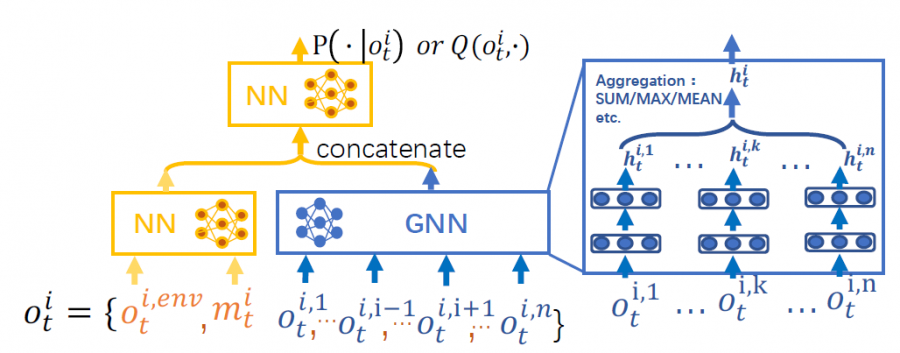## 三、实验内容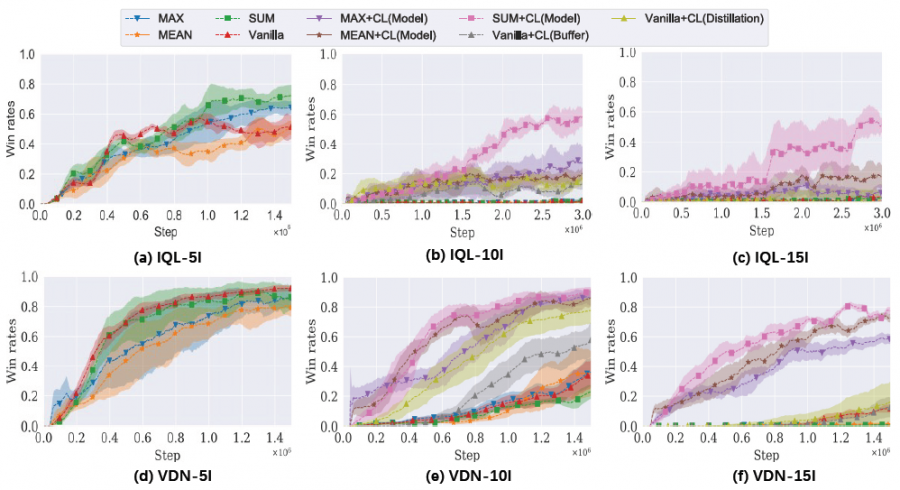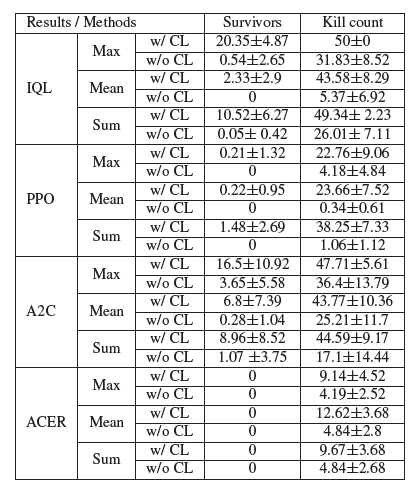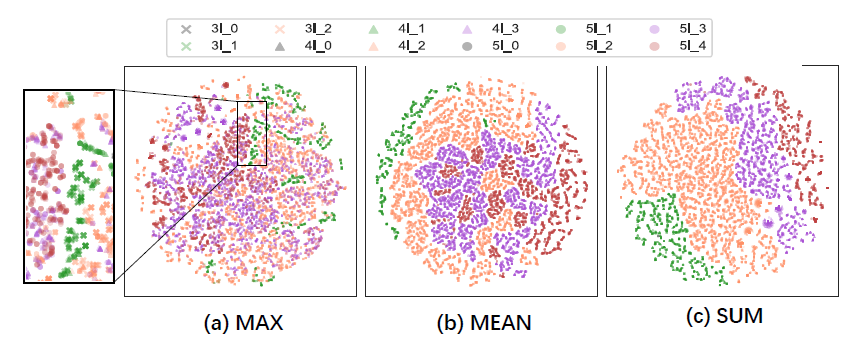## 四、缺点

• 论文本身还是使用了手动设计的课程

## 五、优点

• 论文对于多智能体系统性质的总结非常到位，对于多智能体系统的认知是很好的补充
• 方法具有很直接很可信的insight，有一种大道至简的感觉

##### Share